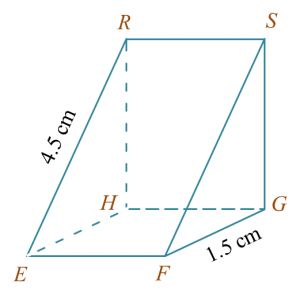# SPM Mathematics 2017, Paper 2 (Questions 1 & 2)

Question 1 (3 marks):
The Venn diagram in the answer space shows set P, set Q and set R such that the universal set ξ = P U Q U R.
(a) P’,
(b) (PQ) U RSolution:
(a)(b)Question 2 (3 marks):
Diagram 1 shows a right prism with a rectangular base EFGH on a horizontal plane. Triangle FGS is the uniform cross section of the prism. GS and HR are vertical edges.Diagram 1

(a)
Name the angle between the plane EFSR and the plane EFGH.
(b) Hence, calculate the angle between the plane EFSR and the plane EFGH.

Solution:
(a)
Angle between the plane EFSR and the plane EFGH
= ∠REH or ∠SFG

(b)